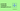# JS Challenge 7: Multiples of 3 or 5In this article we will solve together the Multiples of 3 or 5 challenge from CodeWars, you can find it at this link. The difficulty of this challenge is easy.

If we list all the natural numbers below 10 that are multiples of 3 or 5, we get 3, 5, 6 and 9. The sum of these multiples is 23.

Finish the solution so that it returns the sum of all the multiples of 3 or 5 below the number passed in.

Note: If the number is a multiple of both 3 and 5, only count it once. Also, if a number is negative, return 0(for languages that do have them)

This challenge is very simple and we can achieve the expected result using the remainder operator (`%`).

## The remainder operator

What this oparator does is is return the remainder left over when one operand is divided by a second operand.

Let's look at some examples:

``````6%3;
// 0
6%2;
// 0
6%4;
// 2
6%5;
// 1
6%7;
// 6``````

Let's go over each example:

1) 6%3 = 0 because 3 2 = 6 with no remainder; 2) 6%2 = 0 because 2 3 = 6 with no remainder; 3) 6%4 = 2 because 4 1 = 4 with 2 remainder; 4) 6%5 = 1 because 5 1 = 5 with 1 remainder; 5) 6%7 = 6 because 6 * 0 = 0 with 6 remainder;

Knowing this, we can easily determine if a number is a multiple of 3 or 5 and then perform the sum we need;

## Working on the solution

``````function solution(number){
let sum = 0;
for (var i = 0; i < number; i++) {
if (i % 3 === 0 || i % 5 === 0) {
sum += i;
}
}
return sum;
}``````

1) first we initialise our `sum` variable that will hold the total sum of numbers 2) then we iterate over all the numbers, getting only the one perfectly divisible by 3 or 5, using the `%` (remainder) operator that we saw above 3) lastly we return the sum of all the numbers that match our condition

There are many other ways of solving this problem, let me know yours in the comment.

If you liked this type of content, please let me know in the comments and I'll create more of these.